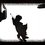# twin prime, am I right or wrong?

I think I proved something, but to be sure I'm right about it, I'm gonna let others trying to prove it (or disprove it) themselves. I'm not so good in all that math symbol thing, so I'm just going to pick a random symbol for all the things here. just go with it please.

let P(n) be the nth prime. for example P(1) = 2, P(2) = 3 , P(4) = 7....

now let Φ(n) = P(1) * P(2) * P(3) ... * P(n)

so what I think I proved is that both Φ(n)+1 and Φ(n) - 1 are primes (twin primes actually).

it's a simple proof, but I think I might be wrong here so I invite anyone who wants to, to prove or disprove it.

hint: you can easily prove that Φ(n) is not divisible by any prime numbers under P(n), but what about prime numbers that are bigger than P(n)?Note by Daniel Magen
6 years, 8 months ago

This discussion board is a place to discuss our Daily Challenges and the math and science related to those challenges. Explanations are more than just a solution — they should explain the steps and thinking strategies that you used to obtain the solution. Comments should further the discussion of math and science.

When posting on Brilliant:

• Use the emojis to react to an explanation, whether you're congratulating a job well done , or just really confused .
• Ask specific questions about the challenge or the steps in somebody's explanation. Well-posed questions can add a lot to the discussion, but posting "I don't understand!" doesn't help anyone.
• Try to contribute something new to the discussion, whether it is an extension, generalization or other idea related to the challenge.

MarkdownAppears as
*italics* or _italics_ italics
**bold** or __bold__ bold
- bulleted- list
• bulleted
• list
1. numbered2. list
1. numbered
2. list
Note: you must add a full line of space before and after lists for them to show up correctly
paragraph 1paragraph 2

paragraph 1

paragraph 2

[example link](https://brilliant.org)example link
> This is a quote
This is a quote
    # I indented these lines
# 4 spaces, and now they show
# up as a code block.

print "hello world"
# I indented these lines
# 4 spaces, and now they show
# up as a code block.

print "hello world"
MathAppears as
Remember to wrap math in $$ ... $$ or $ ... $ to ensure proper formatting.
2 \times 3 $2 \times 3$
2^{34} $2^{34}$
a_{i-1} $a_{i-1}$
\frac{2}{3} $\frac{2}{3}$
\sqrt{2} $\sqrt{2}$
\sum_{i=1}^3 $\sum_{i=1}^3$
\sin \theta $\sin \theta$
\boxed{123} $\boxed{123}$

Sort by:

Well, $\phi(4)-1=209=11\cdot 19$.

- 6 years, 8 months ago

this is a counterexample

- 6 years, 8 months ago

oh well here goes my theory. I hoped it was true, but I guess I knew it had to be wrong. thanks for the counterexample. it actually explains to me why my "proof" was wrong.

- 6 years, 8 months ago

This is not the first time I've seen this.

To be honest, this is the first thing that came to my mind too when I was introduced to the Twin Prime Problem for the very first time.

It turns out that even $\phi(n)+1$ can be composite.

Take $\phi(6)+1=2\times3\times5\times7\times11\times13+1=30031=59\times509$.

- 6 years, 8 months ago

really? I just thought about it for the first time yesterday when I went for a walk, years after I first heard about twin primes. dammit the people on this site are too smart for me.

- 6 years, 8 months ago

Yes, there are some really smart people on this site!

While you proved that all primes up to $P(n)$ don't divide $\phi(n) \pm 1$ [I'm using your notation], you didn't prove that primes larger than $P(n)$ don't divide $\phi(n) \pm 1$.

As $n$ gets larger, $\phi(n) \pm 1$ gets really huge leaving a lot of room for other primes. But I think you now know this.

- 6 years, 8 months ago

"As n gets larger, ϕ(n)±1 gets really huge leaving a lot of room for other primes" yeah you are absolutely right. thanks for taking the time to explain your point of view to me. it really does help to have another person give you his unique point of view.

- 6 years, 8 months ago